# vcjmhg 的个人博客

## 滑动窗口常用技巧总结# 算法与框架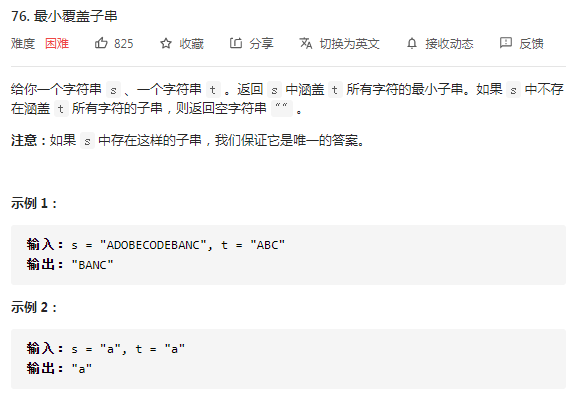1. 在字符串 S 中使用双指针中左右指针的技巧，初始化 `left = right = 0`，把索引区间 `[left,right]` 称之为一个[窗口]。
2. 不断的增加 right 指针扩大窗口 `[left,right ]`，直到窗口中的字符串符合要求（窗口包含 T 中所有字符）。
3. 停止增加 right，转而增加 left 指针，进而缩小窗口直到窗口不再符合要求。同时每增加一个 left 都要更新一轮结果。
4. 重复 2 和 3，直到 right 达到字符串 S 的尽头。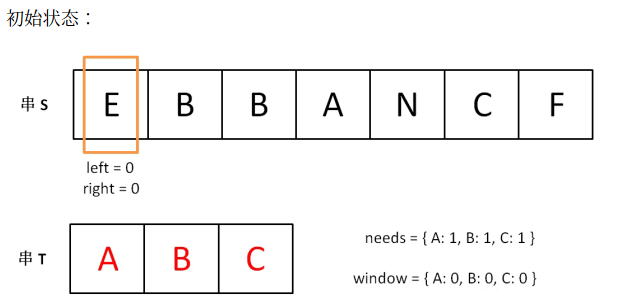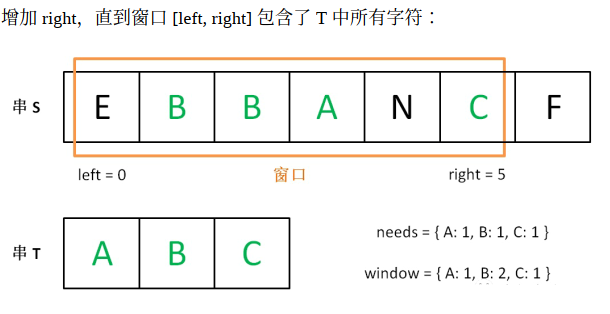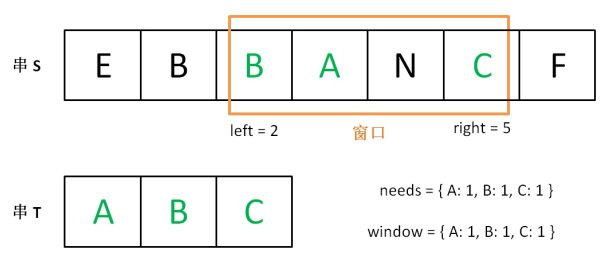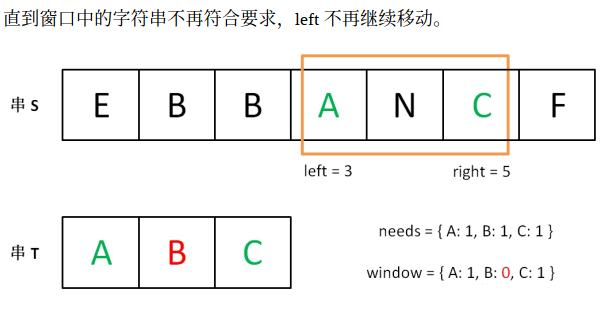`````` 1public String slidingWindow(String s, String t) {
2	//定义两个窗口
3	Map<Character, Integer> need = new HashMap<>(), window = new HashMap<>();
4	// 初始化need窗口
5	for (char c : t.toCharArray()) {
6	  need.put(c, need.getOrDefault(c, 0) + 1);
7	}
8
9	int left = 0, right = 0;
10	// 已经和need匹配的字符串个数
11	int valid = 0;
12	while (right < s.length()) {
13	  char c = s.charAt(right);
14	  // move to right
15	  right++;
16	  // 进行窗口内一系列数据的更新
17	  ...
18
19	// 判断左侧窗口是否要收缩
20	while (window needs shrink) {
21	    // d 是将移出窗口的字符
22	    char d = s.charAt(left);
23	    // 左移窗口
24	    left++;
25	    // 进行窗口内数据的一系列更新
26	    ...
27	}
28}
``````

1. 移动 right 扩大窗口，即加入字符时需要考虑哪些数据？
2. 什么条件下，窗口应该暂停扩大，开始移动 left 缩小窗口？
3. 当移动 left 缩小窗口，即移除字符时，应该更新哪些数据？
4. 我们要的结果应该在扩大窗口时还是缩小窗口时进行更新？

`````` 1string minWindow(string s, string t) {
2    unordered_map<char, int> need, window;
3    for (char c : t) need[c]++;
4
5    int left = 0, right = 0;
6    int valid = 0;
7    // 记录最小覆盖子串的起始索引及长度
8    int start = 0, len = INT_MAX;
9    while (right < s.size()) {
10        // c 是将移入窗口的字符
11        char c = s[right];
12        // 右移窗口
13        right++;
14        // 进行窗口内数据的一系列更新
15        if (need.count(c)) {
16            window[c]++;
17            if (window[c] == need[c])
18                valid++;
19        }
20
21        // 判断左侧窗口是否要收缩
22        //必须使用equlals来判断，不能使用 ==
23        while (valid.equals(need.size())) {
24            // 在这里更新最小覆盖子串
25            if (right - left < len) {
26                start = left;
27                len = right - left;
28            }
29            // d 是将移出窗口的字符
30            char d = s[left];
31            // 左移窗口
32            left++;
33            // 进行窗口内数据的一系列更新
34            if (need.count(d)) {
35                if (window[d].euqals(need[d])
36                    valid--;
37                window[d]--;
38            }
39        }
40    }
41    // 返回最小覆盖子串
42    return len == INT_MAX ?
43        "" : s.substr(start, len);
44}
``````

# 应用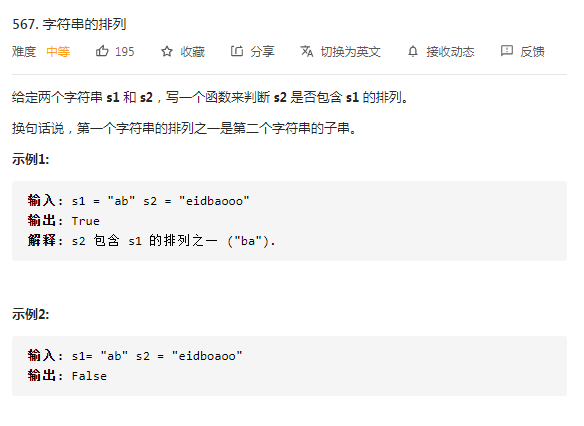1. 右侧窗口滑动时，做哪些操作
2. 左侧窗口滑动的条件，以及所做操作

`````` 1public boolean checkInclusion(String t, String s) {
2    if (t.length() > s.length()) {
3      return false;
4    }
5    Map<Character, Integer> need = new HashMap<>();
6    Map<Character, Integer> window = new HashMap<>();
7    // init need
8    for (char c : t.toCharArray()) {
9      need.put(c, need.getOrDefault(c, 0) + 1);
10    }
11    // define variable
12    int left = 0, right = 0;
13    int valid = 0;
14    while (right < s.length()) {
15      char c = s.charAt(right);
16      right++;
17      // update right window
18      if (need.containsKey(c)) {
19        window.put(c, window.getOrDefault(c, 0) + 1);
20        if (need.get(c).equals(window.get(c))) {
21          valid++;
22        }
23      }
24      // shrink left window
25      // 每一次窗口的尺寸比need的尺寸大的时候都会进行瘦身操作,一直移动到比need的尺寸小1结束
26      while (right - left >= t.length()) {
27        if (valid == need.size()) {
28          return true;
29        }
30        char d = s.charAt(left);
31        left++;
32        if (need.containsKey(d)) {
33          if (window.get(d).equals(need.get(d))) {
34            valid--;
35          }
36          window.put(d, window.get(d) - 1);
37        }
38      }
39    }
40    return false;
41  }
``````